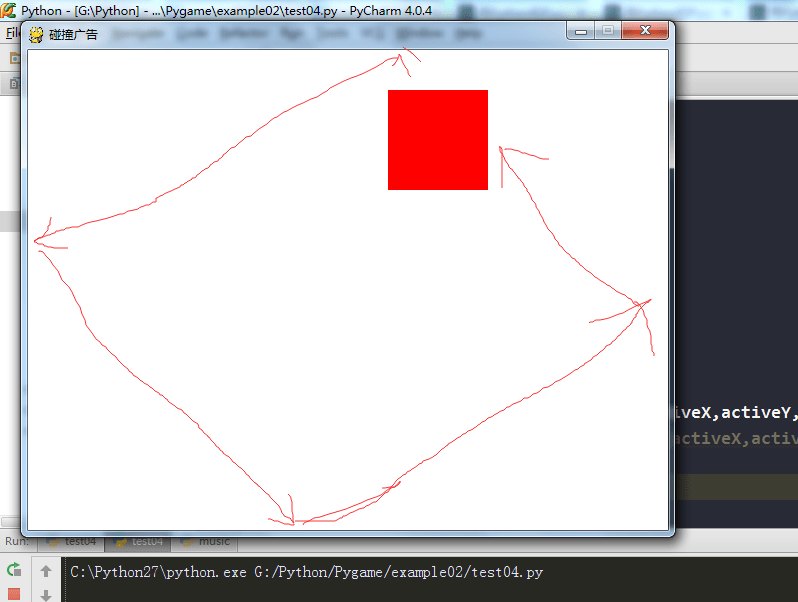#### pygame 做浮动广告~

5-27 3,329 views

# -*- coding:utf-8 -*-
background_image_filename = 'sushiplate.jpg'
sprite_image_filename = 'fugu.png'

import pygame
from pygame.locals import *
from sys import exit

pygame.init()

screen = pygame.display.set_mode((640, 480), 0, 32)

clock = pygame.time.Clock()

x, y = 100., 100.
speed_x, speed_y = 133., 170.

while True:

for event in pygame.event.get():
if event.type == QUIT:
exit()

screen.blit(background, (0,0))
screen.blit(sprite, (x, y))

time_passed = clock.tick(30)
time_passed_seconds = time_passed / 1000.0

x += speed_x * time_passed_seconds
y += speed_y * time_passed_seconds

# 到达边界则把速度反向
if x > 640 - sprite.get_width():
speed_x = -speed_x
x = 640 - sprite.get_width()
elif x < 0:
speed_x = -speed_x
x = 0.

if y > 480 - sprite.get_height():
speed_y = -speed_y
y = 480 - sprite.get_height()
elif y < 0:
speed_y = -speed_y
y = 0

pygame.display.update()centos7安装python3.8

1.安装python 依赖 sudo yum -y install epel-release sudo yum -y update 安装完后可以 reboot 重其服务器,非必须。 2.安装gcc编译环境等等 sudo yum -y gro...

python清空文件夹下log文件

#!/usr/bin/python import os import sys files_list = [] def print_files(path): lsdir = os.listdir(path) dirs = [i for i in lsdir if os.path...

python paramiko（SFTP） 相关文档

python paramiko SFTP文档地址： http://docs.paramiko.org/en/2.4/api/sftp.html import paramiko host = "THEHOST.com" #...